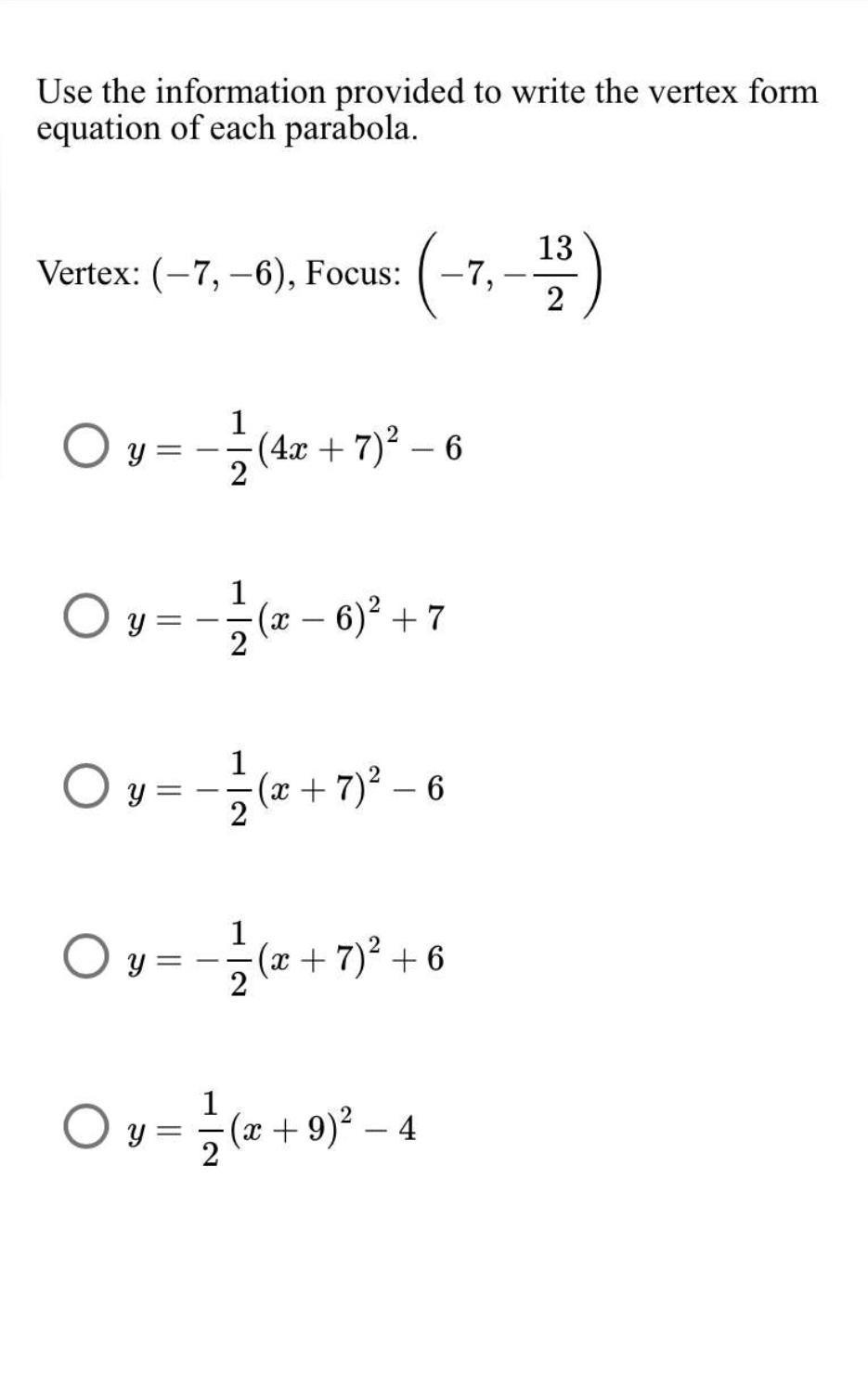Question:

# Use the information provided to write the vertex form equation of each parabola. 13 Vertex: (-7,-6), Focus: (-7, - 2 O y=-(42 +Use the information provided to write the vertex form equation of each parabola. 13 Vertex: (-7,-6), Focus: (-7, - 2 O y=-(42 + 7)2 – 6 1 4x Oy=+*( – 6)2 +7 +(x 2 1 Ov=-(+7)2 - 6 x ? – 2 1 Oy==(x + 7) +6 O y==(x + 9)2 - 4 1 = 2 – .